# How many kilocalories are required to melt 503 g of ice at 0°C. Latent heat of...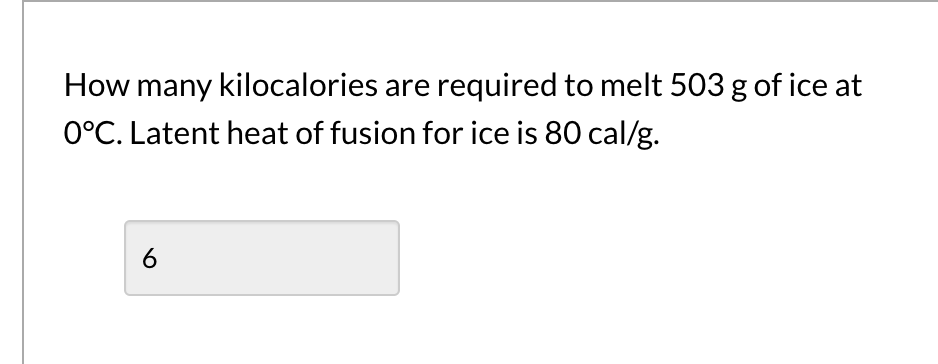How many kilocalories are required to melt 503 g of ice at 0°C. Latent heat of fusion for ice is 80 cal/g. 6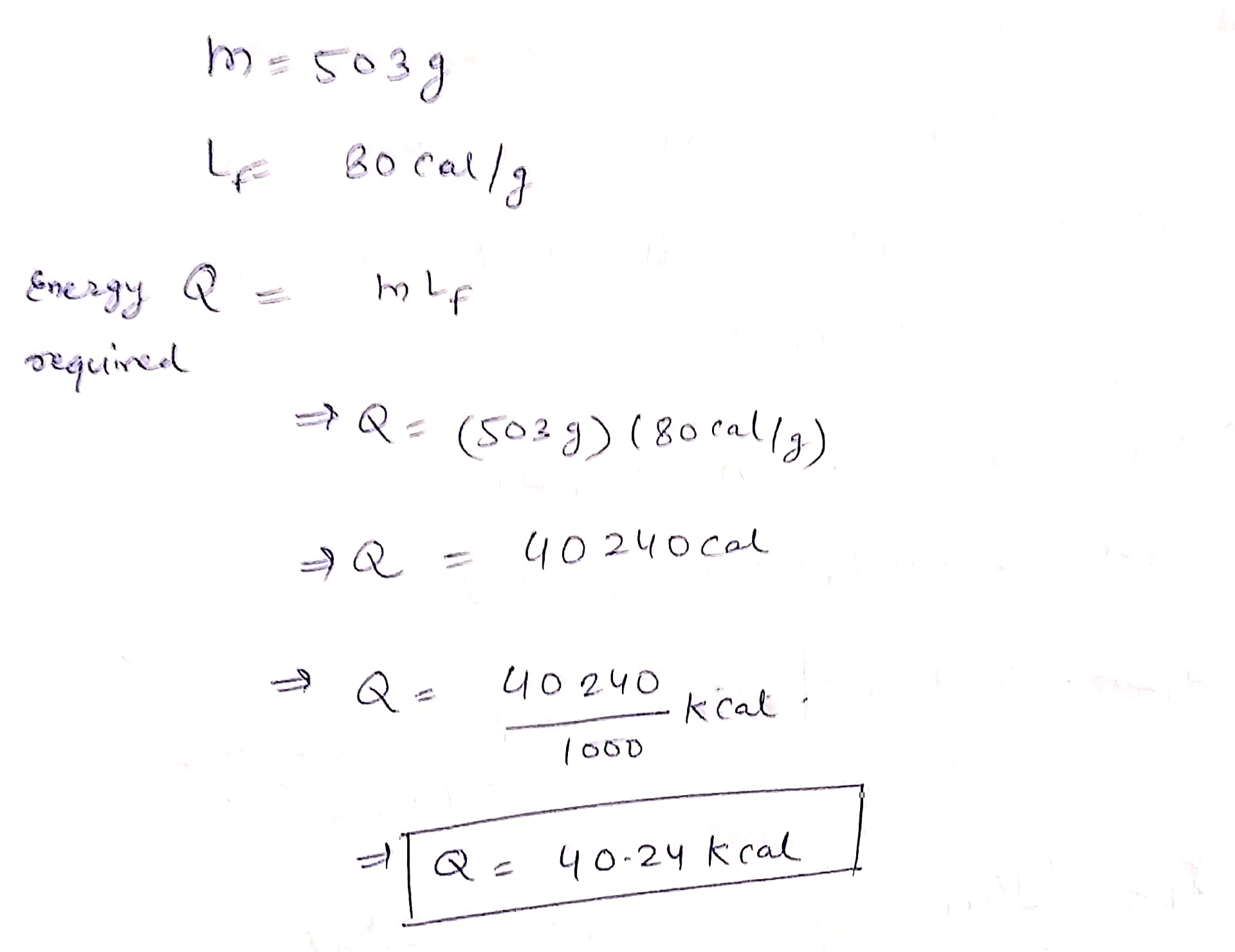##### Add Answer of: How many kilocalories are required to melt 503 g of ice at 0°C. Latent heat of...
Similar Homework Help Questions
• ### The heat of fusion of ice is 80 cal/g. How many calories are required to melt...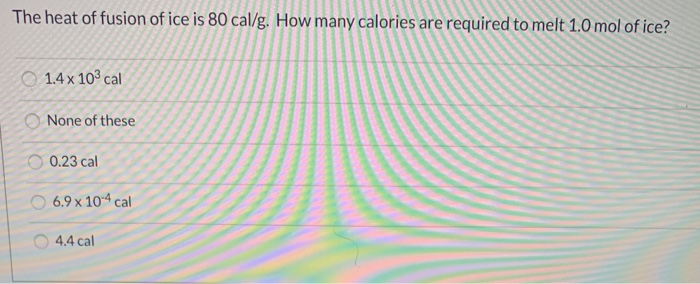The heat of fusion of ice is 80 cal/g. How many calories are required to melt 1.0 mol of ice? 1.4 x 103 cal None of these 0.23 cal 6.9 x 10-4 cal 4.4 cal Show transcribed image text

• ### Problem The latent heat of fusion (solid become liquid) for ice is 79.8 cal/gram

ProblemThe latent heat of fusion (solid become liquid) for ice is 79.8 cal/gram. How much ice can we melt with 1000 calories of heat?ProblemWhich would require more heat, melting 500 g of 0 C ice or turning 500 g of 100 C water into steam?ProblemA 500 g sample of an unknown material requires 750 calories of heat to raise its temperature by 50 C. Use Equation (1) and Table 6.1 to identify the material.

• ### Heat is added to 2.31 kg of ice at -23 °C. How many kilocalories are required...

Heat is added to 2.31 kg of ice at -23 °C. How many kilocalories are required to change the ice to steam at 155 °C?

• ### How many joules of heat are required to melt 40kg of ice at 0 degrees celsious

How many joules of heat are required to melt 40kg of ice at 0 degrees celsious?

• ### 6) There is 15.0 g of ice at 0.0C. How many grams of water at 50.0C must be added to the ice to melt all the ice an...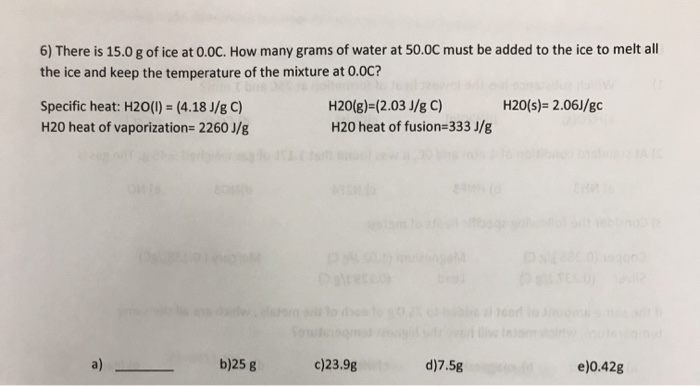6) There is 15.0 g of ice at 0.0C. How many grams of water at 50.0C must be added to the ice to melt all the ice and keep the temperature of the mixture at 0.OC? H2O(s)= 2.06J/gc Specific heat: H2O(0) = (4.18 J/g C) H20 heat of vaporization=2260 J/g H2O(g)=(2.03 J/g C) H2O heat of fusion=333 J/g b)25 8 c)23.98 d)7. 58 )0.428

• ### How many grams of ice will melt?

Fifty grams of ice at 0oC is placed in a thermos bottlecontaining 200g of water at 10oC. How many grams of ice will melt?the heat of fusion of water is 333kJ/kgand the specific heat is 4190J/kg.K.(a) 0(b) 10(c) 25(d) 40(e) 50

• ### How much heat is required to change 456 g of ice at -20.0Degree C into water...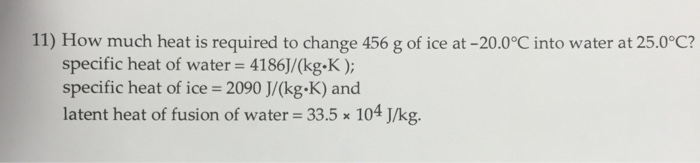How much heat is required to change 456 g of ice at -20.0Degree C into water at 25.0Degree C? specific heat of water = 4186]/(kg-K); specific heat of ice = 2090 J/(kg.K) and latent heat of fusion of water = 33.5 times 10^4 J/kg.

• ### How much heat must be absorbed by ice of mass m = 720 g at -...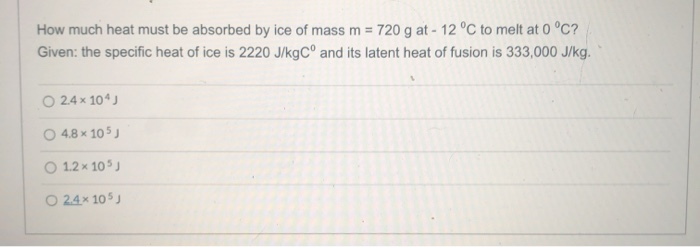How much heat must be absorbed by ice of mass m = 720 g at - 12 °C to melt at 0 °C? Given: the specific heat of ice is 2220 J/kg Cº and its latent heat of fusion is 333,000 J/kg. 24 x 10) 48 x 105) 1.2 x 105) O 24% 105,

• ### What quantity of heat is necessary to convert 50 g of ice at 0 degrees C into steam at 100 degrees C?

The heat of fusion of ice is 80 cal/g., the heat of vaporization of water is 540 cal/g., and the specific heat of water is 1 cal/g.*degrees C.

• ### An ice bag containing 0°C ice is much more effective in absorbing heat than one containing...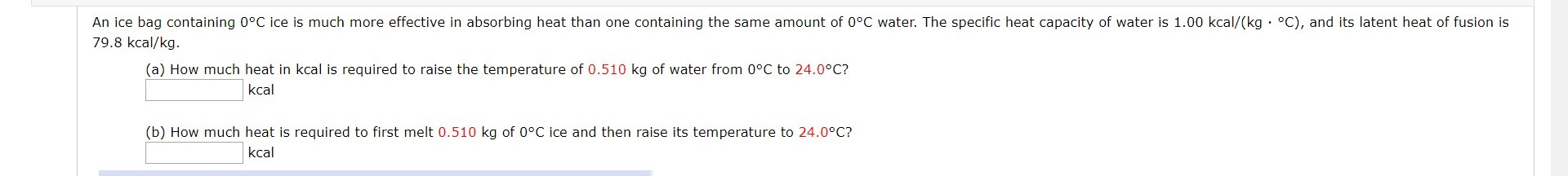An ice bag containing 0°C ice is much more effective in absorbing heat than one containing the same amount of 0°C water. The specific heat capacity of water is 1.00 kcal/(kg . °C), and its latent heat of fusion is 79.8 kcal/kg. (a) How much heat in kcal is required to raise the temperature of 0.510 kg of water from 0°C to 24.0°C? kcal (b) How much heat is required to first melt 0.510 kg of 0°C ice and then...

Free Homework App